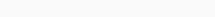# Math Puzzles Puzzle 44 Answer AndroidMath puzzle game level 44 solution with detailed hints and explanation, Scroll below to find out.

Math puzzles increase your logical thinking. The objective of the game is to find patterns hidden in the puzzle in order to arrive at the solution. Challenge yourself with different levels of math puzzles and stretch the limits of your intelligence.

### Math Puzzles Puzzle 44:

1   5   13

29

61  125  ?Solution/Hint:

1+5=6=6*1

5+13=18=6*3

13+29=42=6*7

29+61=90=6*15

61+125=186=6*31

125+?=6*63

If there is any Doubt or you are unable to understand the solution, then please let me know in comments, I will surely help you.

### 8 thoughts on “Math Puzzles Game Level 44 Answer with Solution”

•February 25, 2019 at 23:03

The solution is
5×2+3 = 13
13×2+3 =29
29×2+3 = 61
61×2+3 = 125
125×2+3 = 253

•February 27, 2019 at 03:26

Thank You for providing easy alternate soltion

•April 7, 2020 at 23:18

I did not understood the ans

•April 7, 2020 at 23:19

I did not understood the ans

•February 15, 2019 at 11:24

I can not understand.
125 + α = 6 * 63 How can we find 63?

•February 15, 2019 at 11:26

I can not understand.
125 α = 6 * 63 How can you find 63 in the equation?

•February 15, 2019 at 12:25

I think..
1+5=6=6*1(2*0+1=1)

5+13=18=6*3(2*1+1=3)

13+29=42=6*7(2*3+1=7)

29+61=90=6*15(2*7+1=15)

61+125=186=6*31(2*15+1=31)

125+?=6*63(2*31+1=63)

•